# Non–Verbal Reasoning: Theory & Examples

Non-verbal reasoning questions appear in a number of competitive exams. The questions feature in the form of figures, designs and drawings. This type of reasoning tests your ability to analyse, understand and interpret the visual data and tackle problems using visual reasoning. These are of the following three types:
1. Series
2. Analogies
3. Classification
Let’s discuss each type with the help of examples:
###### Visual Series
Example 1: If the figures continue to change in the same order what should the fifth figure be?”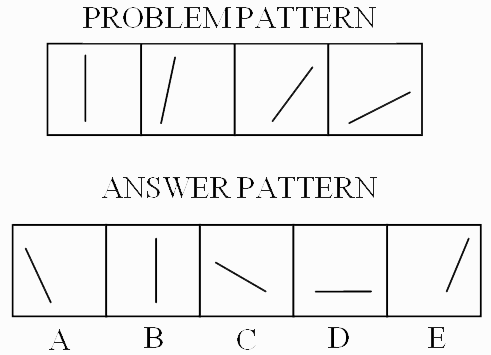Sol: Note that the four figures in the first line are called Problem Pattern. The five figures in the second line are called Answer Pattern. They are indicated by the letters A, B, C, D and E.
The four problem figures together make a series. That means they change, from left to right in a specific order. The question is, “if the figures continue to change in the same order what should the fifth figure be ?"
In the example, as you go from left to right you find that the line in the problem figures is falling down. The question is 'if the line continues to fall, what would be its next, i.e. fifth position. The answer would be: it would fall further and be lying flat i.e. it will be horizontal. Now look at the answer figures. You find that Answer figure D has a line lying horizontal. Therefore, your answer to this problem is D.
Example 2: If the figures continue to change in the same order what should the fifth figure be?”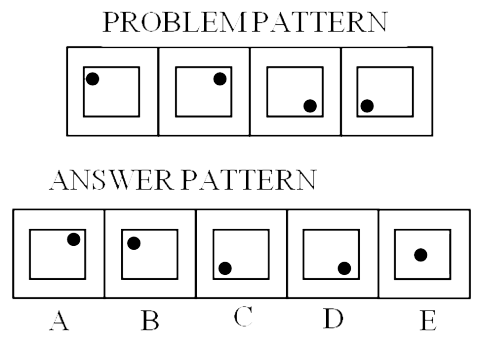Sol: Study the position of the dot in all the problem figures. Note that it keeps moving around the square. It always moves in the clockwise direction. What picture belongs to the next, the fifth square? The dot would come back to the upper corner; therefore, B is the answer.
Example 3: If the figures continue to change in the same order what should the fifth figure be?”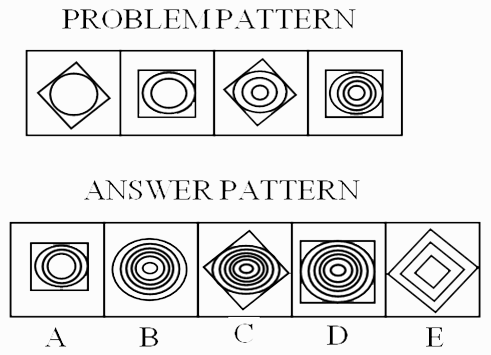Sol: You will see two different things happening here. The number of circles is increasing 1, 2, 3, 4, so that the next figure would have 5 circles. But note that the square is also turning. First the point is up, then the flat side, then again the point is up, and then again the flat side. In the next place, therefore, the point should be up. Only figure C has both: the point is up and, there are circles. So your answer is C.
###### Visual Analogies
Example 4: In the given problem, the first figure is related to the second figure in a certain way. Find the fourth figure, which is related to the third figure in the same way.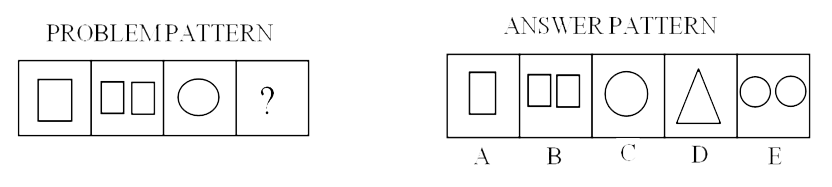Sol: The figures in the first line are -Problem Pattern and those in the second line are Answer Pattern. The problem figures are presented in two units. The first unit contains two figures and the second unit contains one figure and a question mark in place of fourth figure. You have to find out which one of the answer figures should be in place of the question mark. Look at the first two problem figures i.e. the first unit. The first figure is one square and the second figure is two squares. The first figure is related to the second figure in a certain way. That is if first figure is one then the second figure will be double in number but the design would be the same. The third and fourth figures should have the same relationship as the first and second have. That means the fourth figure should be double of the third and the design should be the same for the third and fourth. The third is one circle, so the fourth should be two circles. Figure E shows two circles, so your answer is E.
Example 5: In the given problem, the first figure is related to the second figure in a certain way. Find the fourth figure, which is related to the third figure in the same way.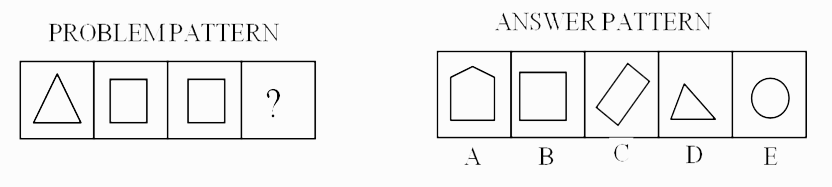Sol: Study the first and the second figures in Problem Figures. The first figure is made up of three lines and the second figure is made up of 4 lines. That means the second figure has one line more than the first figure. The third figure has four lines. Therefore, the fourth figure should have one line more than the third i.e. five lines. Now look at the answer figures. You will find that figure A is made up of five lines. Therefore A is your answer.
Example 6: In the given problem, the first figure is related to the second figure in a certain way. Find the fourth figure, which is related to the third figure in the same way.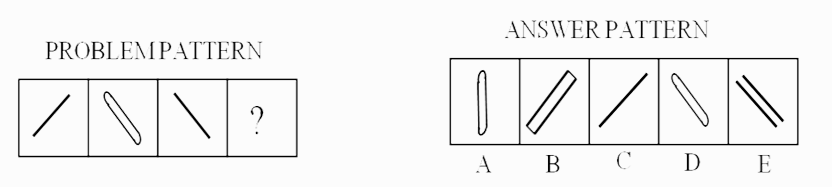Sol: Study the first and the second figures in the problem figures. The two figures are different from each other and the second is inclined in opposite direction of the first. Therefore the fourth figure should be different from the third and inclining in the opposite direction of the third. Such figure is shown by B in answer figures. Therefore B is your answer.
###### Visual Classifications
Example 7: From among the following figures, find the one which is odd one out.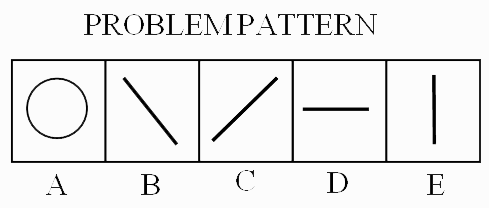Sol: In classification, the problem figures and the answer figures are not separate. There are only problem figures called A, B, C, D and E. Out of these five figures four figures are similar in a certain way. One figure is not like the others. That means four figures belong to one class. The question is which one of the five figures does not belong to this class. Look at the example above. Of the five figures, four are straight lines, whereas one is a circle. The four lines belong to one class. And the circle which is unlike the other figures does not belong to this class. Therefore, your answer to this problem would be the circle, that is A.
Example 8: From among the following figures, find the one which is odd one out.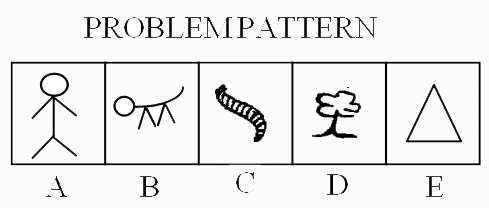Sol: Study the five figures. You find that the first four figures belong to one class, as they represent living being while the fifth – the triangle does not represent a living thing. Therefore, your answer is E
Example 9: From among the following figures, find the one which is odd one out.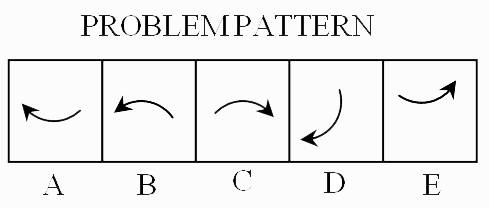Sol: In this problem you find that all the five figures are alike, that is they are curved arrows. But four of the figures i. e. A, B, C, and E are facing either up or down. Only one figure i. e. D faces sideways. Therefore, this figure is different from the other four. Hence your answer is D.
Example 10: Which one of the following patterns does not belong to the class?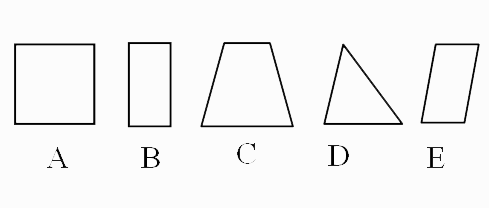Sol: Here all the figures in the group are four sided, except (d) which is a triangle. This is, therefore, different from the others. Hence the answer in this case is (d).
Example 11: Continue the series.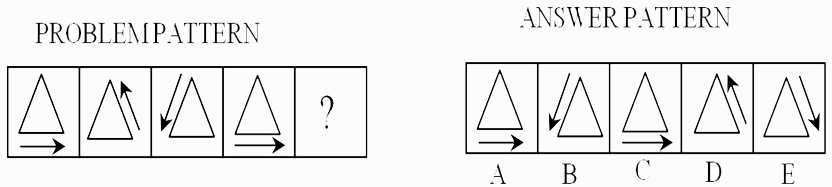Sol: Here the arrows outside the triangle go on moving anticlockwise. Hence the answer pattern in this case will be (d).
Example 12: Continue the series.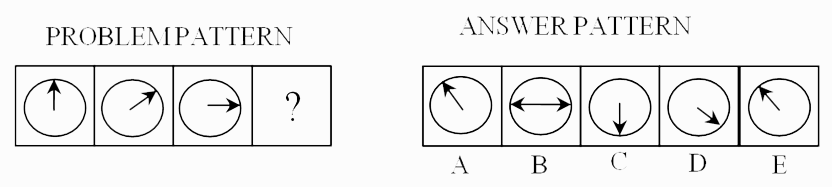Sol: Here the arrow in the circle goes on rotating in clockwise direction at an angle of 45° each time. Hence the answer would be (d).
Example 13: Which of the choices will be next in the series of drawings given below?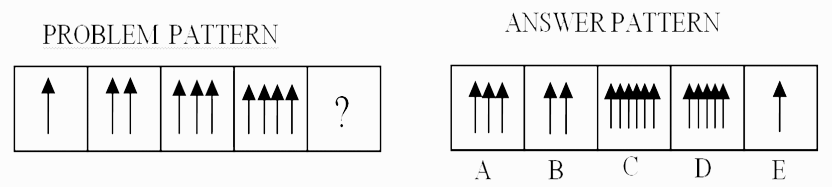Sol: Here the number of arrows goes on increasing one at a time. The answer figure would obviously be that in which there would be five arrows. The answer, therefore, is choice (d).
Example 14: State the number of the choice out of the given answer patterns, which fits into the blank space in the following comparisons:—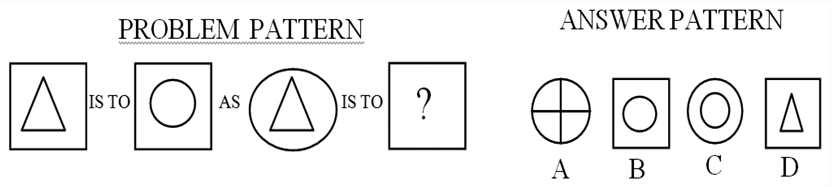Sol: The outer figure in the first two figures is a square. The figure enclosed in each square is different. There is a triangle in the first square and a circle in the second square. There should be same relationship between the third and fourth pattern, that is the outer figure should be the same, in this case a circle; the inner figure in the third figure being a triangle—the inner in the fourth should also be a circle. Hence both the inner and outer figures in the fourth pattern should be a circle. Therefore, the answer in this case is (c).